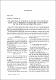## The non-regular 2-groups satisfying the condition: each cyclic subgroup is contained in the center or has a trivial intersection wiyh it2007
Pylyavska, Olha
Shatohina, Ju.
##### Abstract
Y. Berkovich formulated the following problem: «Suppose that p-group (! satisfies the following condition: if C is a cyclic subgroup o f G then either ('< Z(G) or C n Z (C ) = {l}. Classify all such groups.» The authors have proved that each regular p-group satisfies this condition if and only if it is either abelian or a group o f exponent p. Here we regard the non-regular 2-groups. Some necessary conditions for non-abe/ian 2-group in which every cyclic subgroup is contained in the center or has a trivial intersection with it are pointed. The minima! example o f non-regular 2-group with 2 generators satisfying this condition is constructed.
##### Keywords
ZC-групи, мінімальний приклад нерегулярної 2-групи
##### Citation
Pylyavska Olha. The non-regular 2-groups satisfying the condition : each cyclic subgroup is contained in the center or has a trivial intersection wiyh it / O. Pylyavska, Ju. Shatohina // Наукові записки НаУКМА. - 2007. - Т. 61 : Фізико-математичні науки. - С. 11-14.